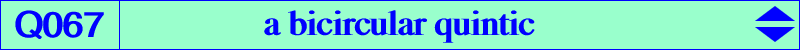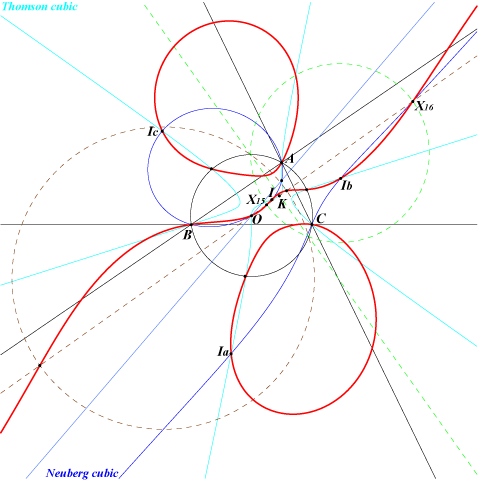too complicated to be written here. Click on the link to download a text file.X(1), X(3), X(15), X(16), X(30) excenters common points of the circumcircle and the Thomson cubicQ067 is a bicircular quintic with one real asymptote which is the Euler line. It is the locus of point P such that the reflection triangle of P is perspective with the symmetric triangle of the isogonal conjugate P* of P. See Table 6. *** This is a special case of a more general class of quintics obtained when the symmetric triangle of P* is replaced by the homothetic triangle T(k) of ABC under the homothety with center P*, ratio k. For each k, this triangle is perspective with the reflection triangle of P if and only if P lies on the circumcircle or on a bicircular quintic Q(k) passing through X(1), X(3), X(15), X(16), X(30), the excenters, the common points of the circumcircle and the Thomson cubic. Obviously, Q067 = Q(2). With k = 1, we find a classical property of the Neuberg cubic (see property 1) since T(1) is ABC itself. The limit cases are also interesting : Q(k->0) is Q037 and Q(k->∞) is decomposed into the line at infinity and Q002, the Euler-Morley quartic. Q(1/2) contains X(187) and its tangent at O is the Brocard axis. Q(-1) contains X(2) and its tangent at O is the line through X(74), X(110). *** Another generalization is obtained when the reflection triangle of P is replaced by P(k), the homothetic of the pedal triangle of P under the homothety with center P, ratio k. P(k) and T(k) are orthologic and the centers of orthology are P and P*. They are perspective if and only if P lies on a circular quintic Q'(k). We have Q'(2) = Q067 and Q'(1) is the union of the circumcircle and the Darboux cubic. The limit case Q'(k->0) gives Q068 and the limit case Q'(k->∞) gives Q069.